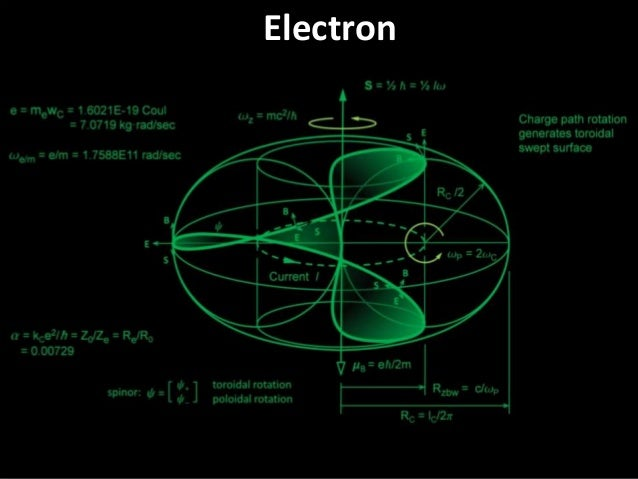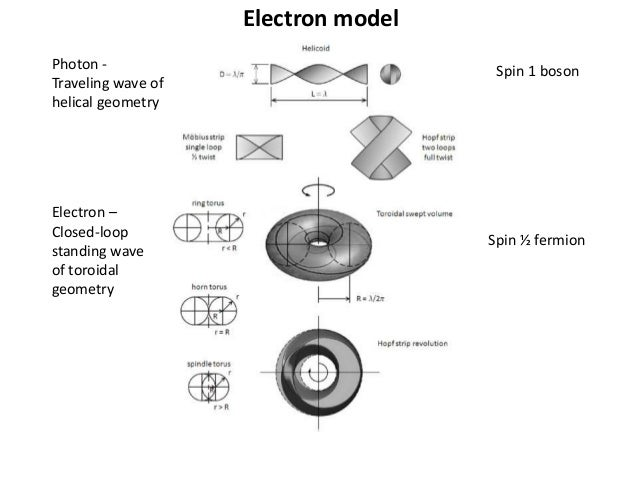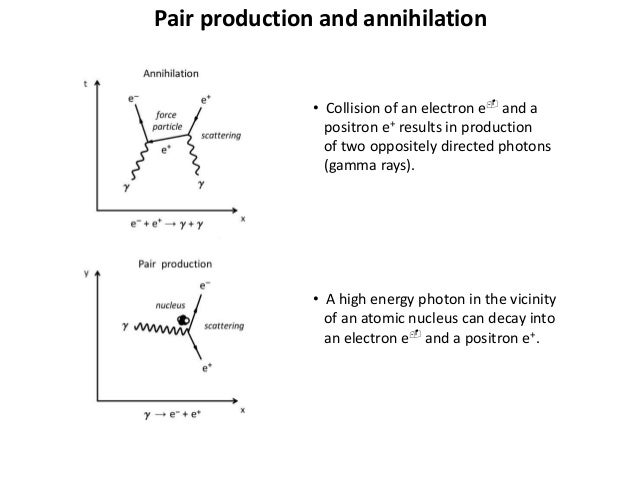Successfully reported this slideshow.Upcoming SlideShare
×

ofUpcoming SlideShare
Next

0 Likes

Share

# Electron

A geometrical model of the electron is shown as a closed-loop standing wave. The charge path is in the form of rotating Hopf link generating a toroidal swept surface with circumference equal to the Compton wavelength. A precessing epitrochoid charge path composed of two orthogonal spinors with toroidal & poloidal current loop components of 2:1 rotary octave resulting in observed 1/2 spin. Electric charge arises a result of a slight precession due to imbalance of electrostatic & magnetostatic energy characterized by a whirl no. equal to the inverse fine structure constant. Quantum mechanical description of electric & magnetic field lines at the Planck scale is depicted.

See all

### Related Audiobooks

#### Free with a 30 day trial from Scribd

See all
• Be the first to like this

### Electron

1. 1. Electron
2. 2. Electron model Photon - Traveling wave of helical geometry Electron – Closed-loop standing wave of toroidal geometry Spin 1 boson Spin ½ fermion
3. 3. Pair production and annihilation • Collision of an electron e- and a positron e+ results in production of two oppositely directed photons (gamma rays). • A high energy photon in the vicinity of an atomic nucleus can decay into an electron e- and a positron e+.
4. 4. Toroidal electron model Parameter Symbol Relation Value Units Electric charge e = F/E = mwC = √(aqP 2) 1.60217E-19 C Mass me = E/c2 = ħc/RC = e/wC 9.10938E-31 kg Compton wavelength lC = h/mc = h/p 2.4263E-12 m Compton frequency fC = mc2/h = c/lC = wC /2p 1.2355E20 Hz Compton radius: RC = lC/2p = aa0 = √(E/mw2) 3.8616E-13 m Compton angular frequency wC = c/RC = mc2/ħ = e/m 7.7634E20 rad/s Zitterbewegung angular frequency wzbw = 2wC 1.5527E21 rad/s Spin angular momentum s = ½ ħ = iw = ½meRC 2w 5.2725E-5 J∙s Bohr magneton mB = e ħ /2m 9.274E-24 J/T Rest Energy E = ħ c/RC 8.187E-14 J • Toroidal electron formed by a high energy photon topologically confined inside the Compton radius. • Propagation of the rotating spin wave describes a current loop equal to ½ of Compton radius. • Charge path rotation generates toroidal swept volume.
5. 5. Electron model cross-section Parameter Symbol Relation Value Units Charge radius Re = √2(lC/4p) = Rzbw/sing 2.7305E-13 m Classical electron radius R0 = (1/4pe0) e2/mec2 = ke(e2/mec2) 2.8179E-15 m Compton radius RC = lC/2p = aa0 3.8616E-13 m Helix radius Rphoton = Rhelix = lC/2p = √(E/me∙w2) 3.8616E-13 m Mass radius (max) Rm = ħc/E = ħ/mc 3.8613E-13 m Poloidal radius Rp = Rm/2 1.9308E-13 m Spindle (inversion) radius Rs = RC – Rm 2.6983E-17 m Toroidal radius Rt = RC(1 + a/2p) 3.8616E-13 m Zitterbewegung radius Rzbw = <Rm> = lC/4p = ħ/2mc = c/2wC = Resing 1.9308E-13 m Chracteristic dimensions • Toroidal circumference equals Compton wavelength lC. • Electron Compton radius equals lC/2p
6. 6. Electron ring configuration • Electron depicted as a precessing epitrochoid charge path composed of two orthogonal spinors of 2:1 rotary octave • Spin ratio of Compton angular frequency wC and Zitterbewegung frequency wzbw (= 2wC) corresponds to observed spin ½. • Electric charge arises as a result of a slight precession of angular frequency we/m.
7. 7. Electron spin precession • Electron spin precession we/m is due primarily to imbalance of electrostatic and magnetostatic energy resulting in an eccentric whirl orbit of the charge path about the spin axis. • Precession follows a zoom-orbit whirl with a periapsis advance q137 that is a function of the fine structure constant a and the Compton radius RC. • Synchronization occurs every a-1 revolutions. Electric charge is a result of a slight spin precession. • Electron mass me = e/wC.
8. 8. Electron toroidal electric field • Electrostatic E-field of an electron is shown time averaged over one rotation period. • For a positron, the electric flux is directed radially inward. • At distances greater than the Compton radius RC, the electric flux distribution for an electron at rest is spherically symmetric equivalent to a point charge.
9. 9. Electron as a rotating spin wave • The electron continuously generates an external dipolar spin wave in the form of a Archimedean spiral rotating at the Compton frequency fC (= 1.236E20 Hz). • The electron acts as a spinning dipole antenna with virtual radiation of a pair of entangled photon wavetrains emitted along the spin axis.
10. 10. Electric field of an oscillating electron • Oscillation of the electron at frequencies less than the Compton frequency fC in response to excitation by an external EM field results in generation of observed EM waves in resonance with the imposed frequency. • Entangled states represent different points on the same wavefront. • Acceleration over time Dt creates a local flux field distortion with the farfield flux pointing in direction of the retarded initial starting position.
11. 11. Electron toroidal magnetic field • Magnetostatic B-field of an electron is shown time averaged over one rotation period. • External magnetic field is toroidal while the internal field is poloidal. • Magnetic flux is concentrated in the central region with increased potential magnetic energy.
12. 12. Electron represented schematically as a primative electrical machine
13. 13. Electron energy storage • During acceleration of an electron, kinetic energy is stored in the magnetic field. • The radiation field dissipates during and subsequent to electron deceleration as the electromagnetic field regains symmetry.
14. 14. Electrostatic & Magnetostatic energy vs. Velocity ratio b Variation of electron energy as a function of velocity ratio b (= v/c)
15. 15. Electromagnetic energy E vs. Lorentz factor g After Bergman The Lorentz factor g is inversely proportional to the Lorentz contraction g. g = 1/√(1 – v2/c2) = 1/√(1 – b2) = 1/g Electromagnetic energy of an electron as a function of Lorentz factor g.
16. 16. Electron Compton radius RC vs. Lorentz factor g • Absorption of energy causes electrons to contract in size increasing the wave function curvature, kinetic energy and volumetric energy density. Variation in electron radius as a function of Lorentz factor g (= 1/√(1 – b2)
17. 17. Electron Compton radius RC vs. Velocity ratio b Variation in electron radius as a function of velocity ratio b (= v/c) • The Compton radius reflects an equilibrium between torsion and the gravitomagnetic field. Contraction in radius occurs as spin remains constant and torsion decreases accordingly.
18. 18. Electron mass energy MeV/c2 vs. Velocity ratio b Relativistic increase in electron mass energy as a function of velocity ratio b (= v/c)
19. 19. Electron Inductance L & Capacitance C vs. Velocity ratio b Relativistic variation in electron inductance L and capacitance C as a function of velocity ratio b (= v/c = Df/p = r/g)
20. 20. Electron wave-function eigenstates in a deep harmonic oscillator 2D potential well • Electron represented as a resonant spin density wave confined in an oscillating deep potential well in a quantum vacuum. • Zitterbewegung corresponds to the motion of the center of charge around the center of mass with a frequency twice the Compton frequency.
21. 21. Quantum electrodynamics (QED) diagrams
22. 22. Electron/positron pair production Electron Compton wavelength, zitterbewegung wavelength, and de Broglie wavelength compared to the wavelength of an energetic photon required for pair production of an electron e- and positron e+.
23. 23. Energy diagram electron/positron pair production
24. 24. Electron/positron production from an energetic photon
25. 25. Electron Coulombic repulsion • Maximum Coulomb repulsive force between electrons occurs at closest proximity equal to a separation distance ar Compton’s radius RC. • The radiated EM wavefronts are in the form of of Archimedean spiral forms. Two electrons on approach are repelled by constructive EM wavefront interference. • Positron spin waves rotate in a direction opposite to electrons. Electron and positron interaction give rise to destructive interference.
26. 26. Spin wave precession • Electric charge is intrinsic to matter and exhibits similarities to topological and vortical charge. • Electric charge of the electron has dimensions of spin angular momentum and appears to be the result of a slight precession equal to the inverse fine structure constant a-1.
27. 27. Elementary Particles of Matter Mass, charge and spin characteristics of fundamental particles and anti-particles
28. 28. Electric and color charge symmetry • When there is symmetry, there is conserved charge. • Symmetry alone does not provide a dynamical origin of charge or define underlying fundamental dimensionality
29. 29. Electric charge vs. topological charge • Geometrical relation of electric charge (e-, e+) to topological charge F is illustrated in the form of a Tusci couple (2-cusp hypocycloid) which corresponds to Special Unitary Group SU(2)
30. 30. Spinor representation of ½ spin characteristic Spinor examples of 720 degree rotation to return to initial orientation involving two rotational frequencies differing by a factor of 2.
31. 31. Quark color charge Twist ribbon represention of quark color charge interactions and equivalent 3-phase circuit
32. 32. Neutral Pi-meson (Pion) • Neutral Pion p0 consists of a spin 0 neutral pi-meson composed of positron-electron pair (Sternglass model) • The positron and electron pair each have spin angular momentum oriented either parallel or anti-parallel to each other. • The orbital angular momentum is equal to twice the spin angular momentum (L = ħ). A spin 0 neutral pion has a life- time of ~0.83E-16 sec and can decay into gamma rays. • Binding energy is decreased when magnetic moments are parallel due to mutual repulsion.
33. 33. Positively charged Mu-meson (Muon) • Positive Muon m+ consists of a positron-electron pair and orbital positron (Sternglass model) • Positron e+ is at rest relative to the precessing frame KP of the central pair system consisting of a spin 1 neutral pi-meson p0 1.
34. 34. Positively charged Pi-meson (Pion) • Positive pion p+ consisting of a positron-electron pair and orbital positron (Sternglass model) • A positron e+ is in orbital motion relative to the precessing frame Kp of a central pair system consisting of a spin 1 neutral pi-meson p0 1.
35. 35. Negatively charged Tau-meson (Tauon) • Negative Tauon t- consisting of a positron-electron pair and orbital electron (Sternglass model). • Electron e- is in orbital motion relative to the precessing frame Kp of the central pair system consisting of a spin 1 neutral pi-meson p0 1.
36. 36. Gluon field Fresnel zone pattern • In-phase and out-phase gluon field Fresnel zone patterns with Airy diffraction cross- section. • Quark separation in a proton with Compton radius RCp of 2.1031E-16 m suggest a gluon field with a minimum distance of ~0.33E-15 m or ~1.56 RCp.
37. 37. Electron-positron coaxial wave interference • Coaxial coupling of an e-e+ pair with aligned spins as a hypothetical quark model with a massless spin 1 field. • A charge screen formed by depolar- ized Planck mass dipoles prevents Coulombic attraction. • The electron and positron oscillate along the spin axis alternating between a state where charge screening is effective at close distance and further out where charge screening is ineffective thus exhibiting asymptotic freedom.
38. 38. Quantum Chromodynamic models Quantum chromodynamics (QCD) representations of protons and neutrons shown as quark composites
39. 39. Proton model • A proton composed of electrons and positrons equivalent to QCD quark representation. • Proton absorption of an antineutrino results in transformation into a neutron with emission of a positron and neutrino in inverse beta decay. • The bulk of the proton’s mass is due to kinetic energy of component quarks.
40. 40. Gravitational force on electron In free fall, the acceleration of gravity and associated frequency difference is equal to zero
41. 41. Electric flux lines • Faraday electric flux lines fE are postulated to be an effect of synchronous alignment of positive and negative Planck mass dipoles of the quantum vacuum. • Alignment results in tension along the flux lines due to attraction of positive and negative masses and compression perpendicular to the flux lines. • Electric field intensity E is a function of the product of the Planck electric dipole moment m0P and the Planck mass mP.
42. 42. Magnetic flux lines • Magnetic flux lines fB are interpreted as vortex filaments of rotating Planck mass dipoles. Magnetic field lines are the result of spin axis alignment of multiple dipoles. • Magnetic field intensity H is a measure of the vortex strength of entrained dipoles • Photons and electrons are spin waves in the Planck quantum vacuum composed of positive and negative Planck masses. Photons are traveling waves. Electrons are standing waves.
43. 43. Electron vacuum resonance Electron and Planck vacuum resonance characterized by lP /lC ratio
44. 44. Abstract A comprehensive description of the nature of light, electricity and gravity is provided in terms of quantum wave mechanics. Detailed models include the photon as a travelling electromagnetic wave and the electron as a closed loop standing wave formed by a confined photon. An electron is modeled as a torus generated by a spinning Hopf link as a result of an imbalance of electrostatic and magnetostatic energy. Electric charge is a manifestation of a slight precession characterized by the fine structure constant. The physical vacuum as a polarizable medium enables wave propagation and appears ultimately to be quantized at the Planck scale. Standing wave transformations for objects in motion are reviewed and Lorentz Doppler effects compared. The mechanism for generation De Broglie matter waves for objects in motion is depicted including the inverse effect of induced motion of an object by synthesis of contracted moving standing waves. Gravity is viewed as a frequency synchronization interaction between coupled mass oscillators. The acceleration of gravity is described by a spectral energy density gradient. Antigravity corresponds an inversion of the naturally occuring energy density gradient. Gravitons are shown to be phase conjugate photons. The metric of curved spacetime corresponds to the electromagnetic wave front interference node metric. Hence, the gravitational field becomes quantized. Quantum Wave Mechanics
45. 45. To order print copies of this book, contact: https://booklocker.com/10176 https://booklocker.com/books/10176.html or https://www.amazon.com/Quantum-Wave- Mechanics-Larry-Reed/ dp/16349249640

Total views

474

On Slideshare

0

From embeds

0

Number of embeds

0

19

Shares

0